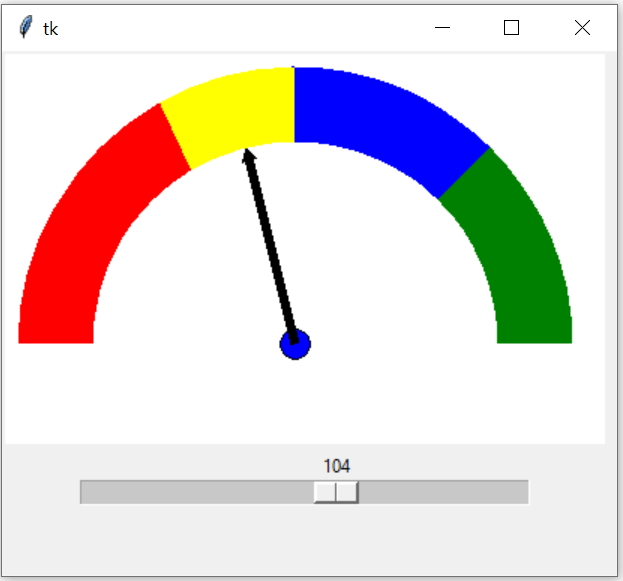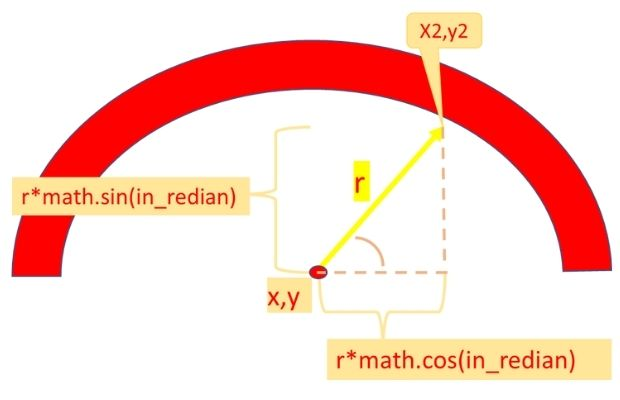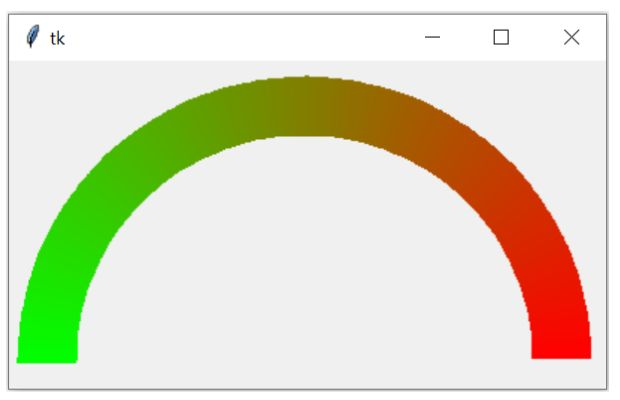# Scale value as Arc slider in CanvasTkinter dashboard to show value of slider of a scale using pointer and region of arcs in Canvas

Scale value can change from 0 to 180
On change of scale slider the function my_upd() will be executed. Inside this function the slider value is collected and converted to radian angle value. Using sin() and cos() functions the needle position is calculated. Old position of needle is deleted and fresh line is created using new coordinates.

## Drawing Arc with pointer

As we have used 4 segments, so we will draw four arc having common x1,y1,x2,y2 coordinates but different start angle and extent. We will give different outline colour to each arc segments.
``````c1.create_arc(x1, y1,x2,y2, start=0, extent=45,outline='green',
width=arc_w,style=tk.ARC)
c1.create_arc(x1, y1,x2,y2, start=45, extent=45,outline='blue',
width=arc_w,style=tk.ARC)
c1.create_arc(x1, y1,x2,y2, start=90, extent=30,outline='yellow',
width=arc_w,style=tk.ARC)
c1.create_arc(x1, y1,x2,y2, start=120, extent=60,outline='red',
width=arc_w,style=tk.ARC)``````
We will add one circle at the center point.
``c1.create_oval(x-10,y-10,x+10,y+10,fill='blue')``

## Drawing PointerOur pointer initial position will be at 0 degree. We have to use radian as input angle to our sin() or cos() functions.
``````in_radian=math.radians(0) # getting radian value from zero degree
# Initial position of pointer at 0 degree

## Moving the Pointer

To move the pointer we will draw it at different positions based on the scale slider value. This value we will use with sin() and cos() functions and then get the end point or x2, y2 values of the pointer. Each time we draw the pointer, we delete the previous pointer.
``````def my_upd(*args): # updating the pointer position
global pointer
c1.delete(pointer) # delete the pointer
Full code is here .
``````import tkinter as tk
import math
my_w = tk.Tk()
width,height=410,310 # set the variables
d=str(width)+"x"+str(height+40)
my_w.geometry(d)
c1 = tk.Canvas(my_w, width=width-10, height=height-50,bg='#FFFFFF')
c1.grid(row=0,column=0)
arc_w=50 # width of the arc
x1,y1,x2,y2=35,35,355,355 # dimensions for the arc
x,y,r=195,195,135

c1.create_arc(x1, y1,x2,y2, start=0, extent=45,outline='green',
width=arc_w,style=tk.ARC)
c1.create_arc(x1, y1,x2,y2, start=45, extent=45,outline='blue',
width=arc_w,style=tk.ARC)
c1.create_arc(x1, y1,x2,y2, start=90, extent=30,outline='yellow',
width=arc_w,style=tk.ARC)
c1.create_arc(x1, y1,x2,y2, start=120, extent=60,outline='red',
width=arc_w,style=tk.ARC)

# small circle at center
c1.create_oval(x-10,y-10,x+10,y+10,fill='blue')

def my_upd(value):
global line
c1.delete(line) # delete the pointer

my_scale = tk.Scale(my_w, from_=0, to=180, orient='horizontal',
length=300,command= my_upd)
my_scale.grid(row=2,column=0)
my_w.mainloop()``````

## Creating continuous Arc colour``````import tkinter as tk
my_w = tk.Tk()
width,height=400,220 # set the variables
d=str(width)+"x"+str(height)
my_w.geometry(d)
my_c = tk.Canvas(my_w,width=width,height=height)
my_c.pack()
x1,y1,x2,y2=25,30,370,370
arc_w=40
def my_upd(d):
change=int((255/180)*d) # Jump in colour value
color_c='#%02x%02x%02x' % (255-change, change,0)
return color_c
res=1 # resolution or steps
for d in range(0,180,res):
my_c.create_arc(x1, y1,x2,y2, start=d, extent=res+1,outline=my_upd(d),
width=arc_w,style=tk.ARC)
my_w.mainloop()``````

Subscribe to our YouTube Channel here

## Subscribe

* indicates required
Subscribe to plus2netplus2net.com